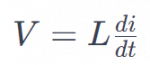# Inductance in Electricity: What It Is, Behavior and ImportanceInductance is one of the fundamental concepts in the field of electricity and electronics. It is a property that manifests itself in electrical circuits and has a significant impact on their behavior.

In this article, we will explain in detail what inductance is in electricity, how it works and its importance in various applications.

## What Is Inductance?

Inductance is an electrical property that manifests itself in a component called an inductor. An inductor is a passive device used in electrical circuits to store energy in the form of a magnetic field. It consists of a coil of wire wound around a core, which can be made of different materials, such as iron or air.

When an electric current is applied to an inductor, it generates a magnetic field around itself.

The intensity of this magnetic field depends on the current flowing through the inductor and its geometric characteristics, such as the number of turns in the coil and the type of core used.

## Inductance Behavior

Inductance is measured in henries (H) and is a measure of the opposition that an inductor presents to the change in current flowing through it. This property derives from the fact that a change in current in an inductor generates an induced electromotive force, according to Faraday's law of electromagnetic induction.

When you try to change the current flowing through an inductor, it opposes the change, resulting in a back electromotive force (EMF) that acts to keep the current constant.

Mathematically, this is expressed by the following equation:Where:

• V is the induced electromotive force (EMF) in volts (V).

• L is the inductance in henries (H).

• di / dt is the rate of change of current in amperes per second (A/s).

This equation shows that the induced EMF is directly proportional to the rate of change of current. The greater the inductance, the greater the opposition to the change in current.

## Importance of InductanceInductance plays an essential role in numerous electronic and electrical applications. Some of the areas where inductance plays a crucial role are as follows:

• Signal Filtering : Inductors are used in filtering circuits to block or allow certain signal frequencies to pass through. This is essential in the construction of stabilized power supplies and radio frequency circuits.
• Energy Storage : Inductors can store energy in their magnetic field and release it later into the circuit. This is used in applications such as power converters and battery charging and discharging systems.
• Interference Suppression : In electronic circuits, inductors are used to suppress electromagnetic interference and maintain signal integrity.
• Transformers : Transformers, which are based on inductance principles, are essential for changing alternating currentvoltage in power supply and power distribution applications.
• Magnetic pulse generation : Inductors are also used in the generation of magnetic pulses in applications such as automotive engine ignition and radio communication systems.
• Solar energy : the conversion of solar energy into electricity through electromagnetic induction is the basis of the operation of photovoltaic panels used to harness solar energy.
Author:

Published: October 2, 2023
Last review: October 2, 2023